# R语言实现决策曲线分析

```library(devtools)
install_github("mdbrown/DecisionCurve")```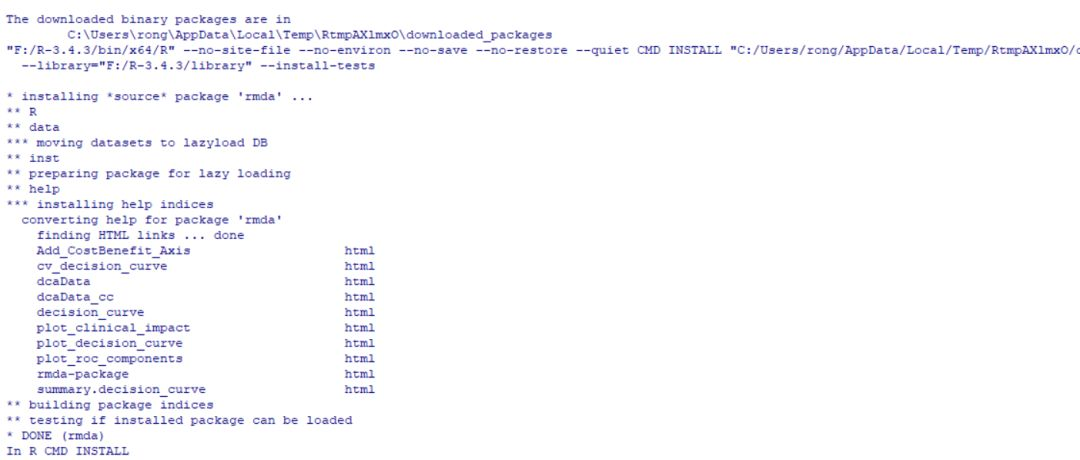1. decision_curve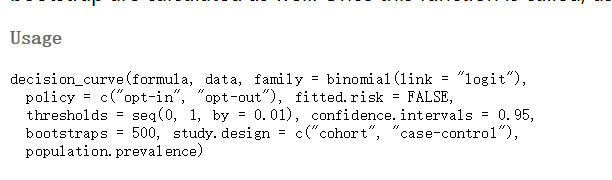formula主要是因变量和自变量的变量名称设置，中间用~区分开。当然在这里因变量都是二进制数据。

2. summary.decision_curve 等同于summary列出评估模型的所有内容

3. plot_decision_curve 绘制以上生成的决策曲线

4. plot_clinical_impact 绘制每个计算点的样本数量。主要是查看真实分布和模型预测分布之间的差异或者说是否一致。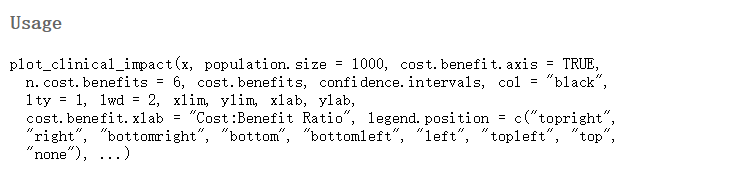5. plot_roc_components绘制ROC的假阳性和真阳性的概率分布。

6. cv_decision_curve以交叉验证方式构建评估模型。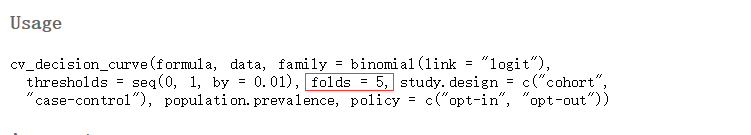```data(dcaData)baseline.model <- decision_curve(Cancer~Age + Female + Smokes,   data = dcaData, thresholds = seq(0, .4, by = .01), study.design = 'cohort',  bootstraps = 10)

full.model <- decision_curve(Cancer~Age + Female + Smokes + Marker1 + Marker2, data = dcaData,thresholds = seq(0, .4, by = .01), bootstraps = 10)```

`summary(baseline.model)`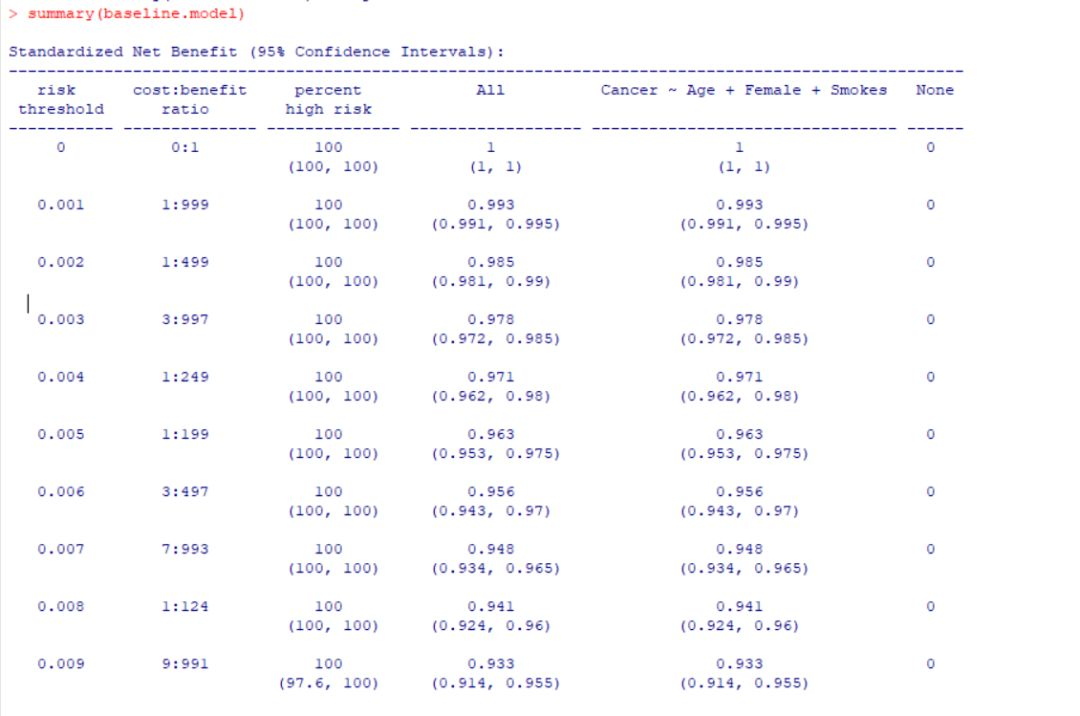`plot_decision_curve( list(baseline.model, full.model), curve.names = c("Baseline model", "Full model"),col = c("blue", "red"),lty = c(1,2),                    lwd = c(3,2, 2, 1),legend.position = "bottomright")`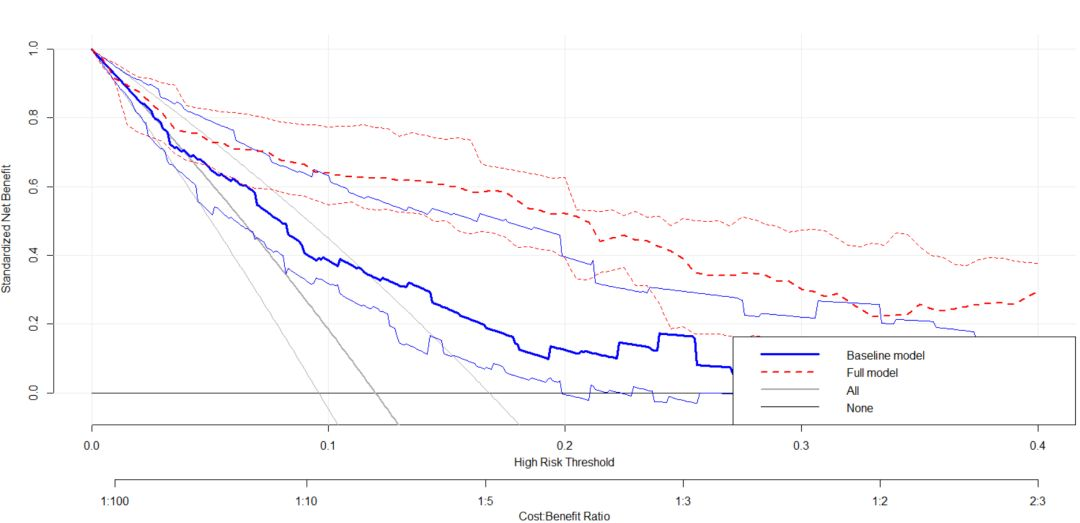```plot_decision_curve( list(baseline.model, full.model),  curve.names = c("Baseline model", "Full model"),  col = c("blue", "red"),

confidence.intervals = FALSE,  #remove confidence intervals

cost.benefit.axis = FALSE, #remove cost benefit axis

legend.position = "none") #remove the legend```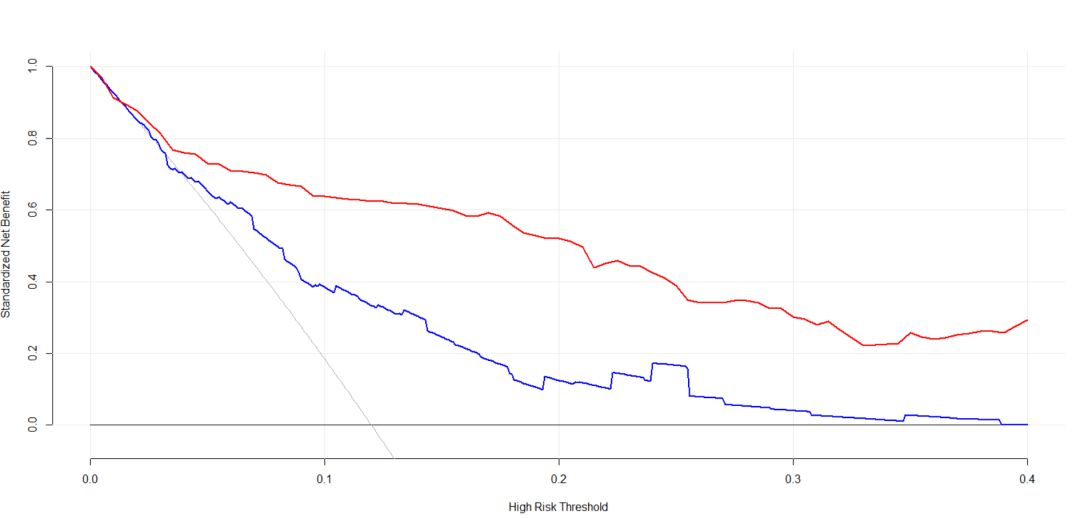`plot_clinical_impact(baseline.model, xlim = c(0, .4),  col = c("black", "blue"))`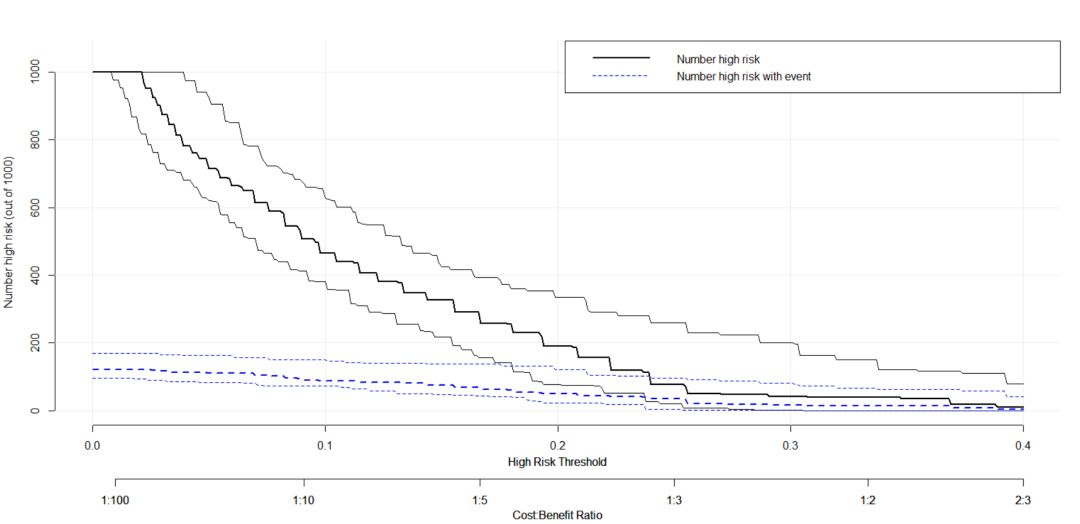`plot_roc_components(baseline.model,  xlim = c(0, 0.4), col = c("black", "red"))`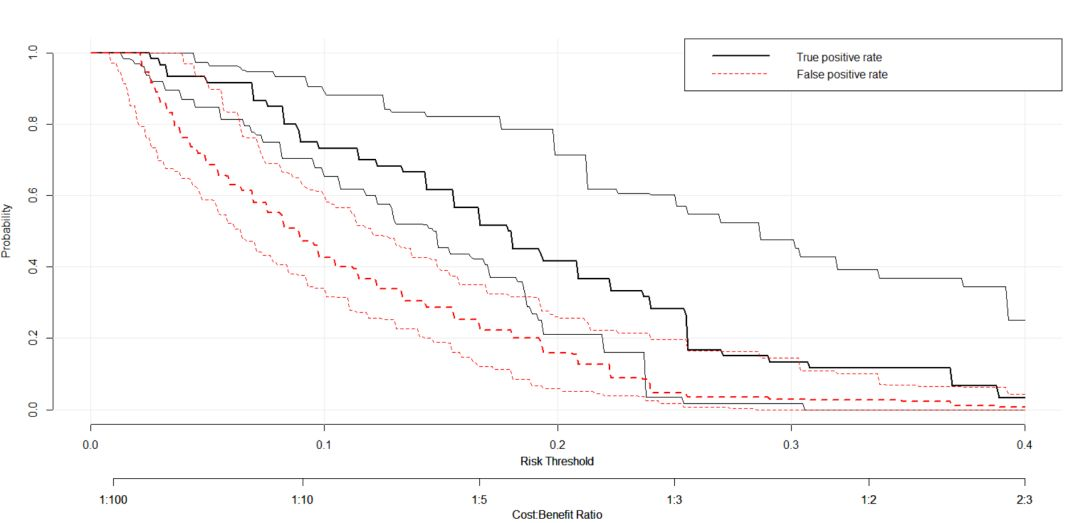```full.model_cv <- cv_decision_curve(Cancer~Age + Female + Smokes + Marker1 + Marker2, data = dcaData, folds = 5, thresholds = seq(0, .4, by = .01))

plot_decision_curve( list(full.model_apparent, full.model_cv), curve.names = c('Apparent curve', 'Cross-validated curve'), col = c('red', 'blue'),lty = c(2,1),     lwd = c(3,2, 2, 1), legend.position = 'bottomright')```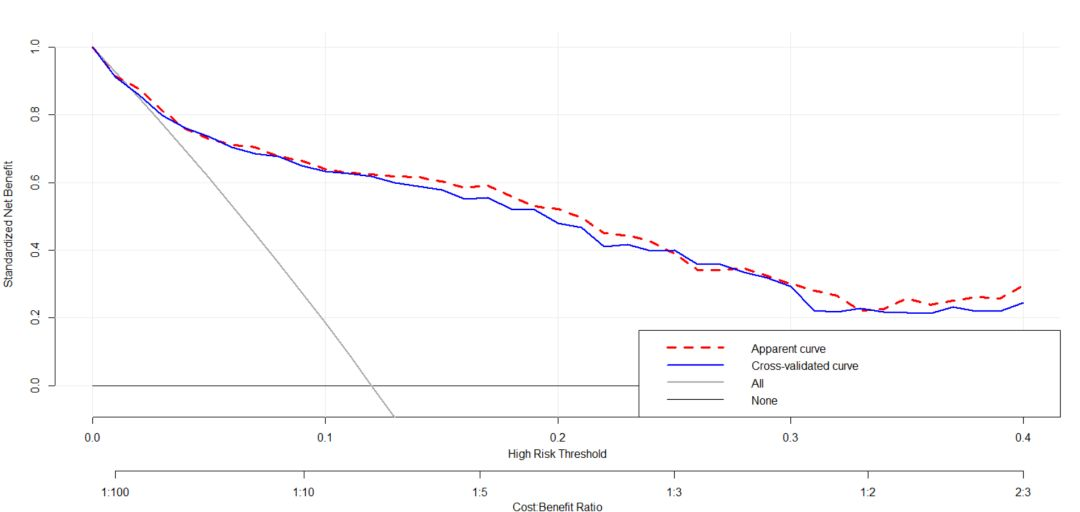• 版权声明 本文源自 R语言交流中心 整理 发表
• 转载请务必保留本文链接：https://www.plob.org/article/22293.html